In our last post, we started building the intuition around constructing a reasonable portfolio to achieve an acceptable return. The hero of our story had built up a small nest egg and then decided to invest it equally across the three major asset classes: stocks, bonds, and real assets. For that we used three liquid ETFs (SPY, SHY, and GLD) as proxies. But our protagonist was faced with some alternative scenarios offered by his cousin and his co-worker; a Risky portfolio of almost all stocks and a Naive portfolio of 50/50 stocks and bonds.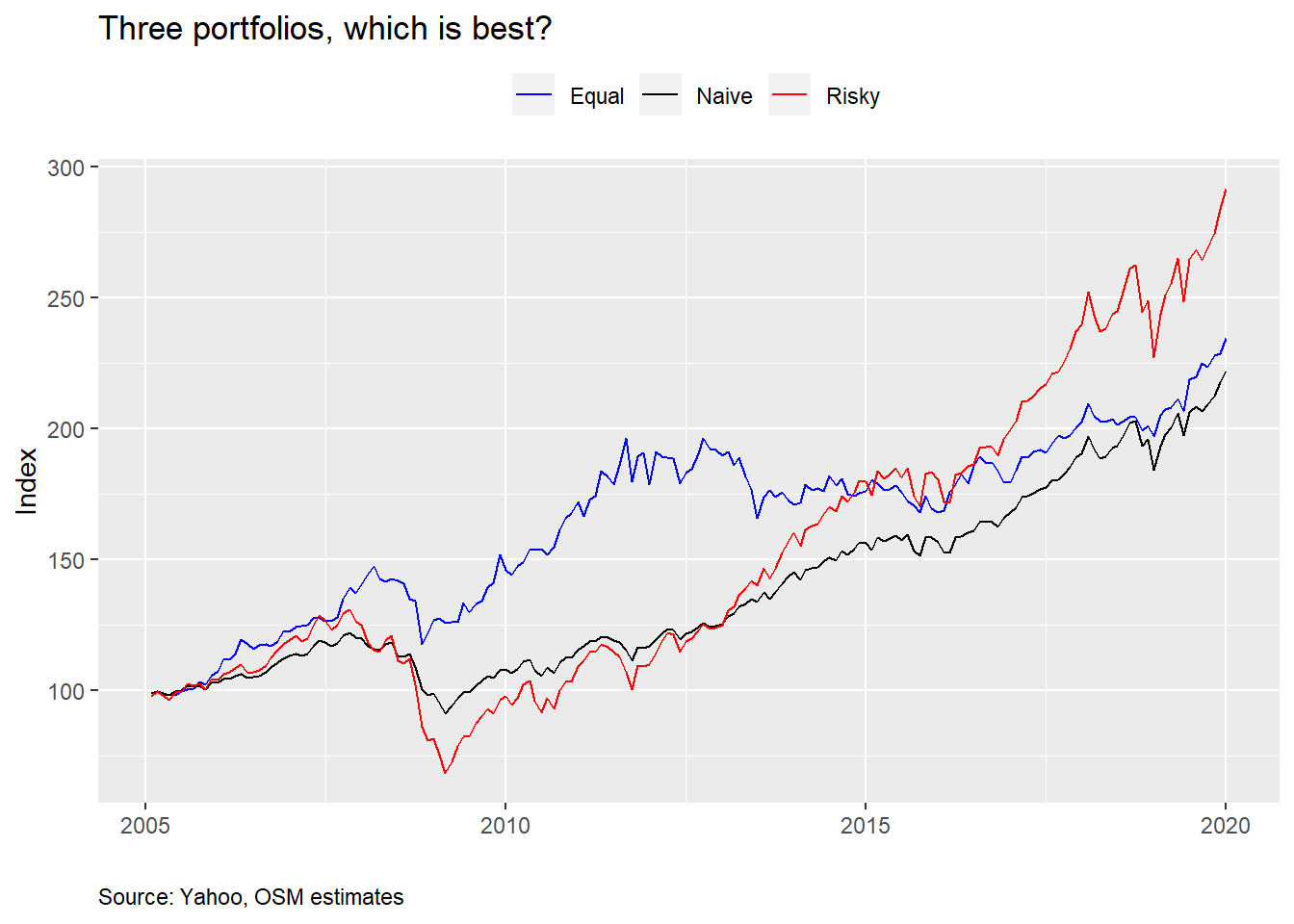After seeing the outcomes of the different portfolios, our hero wondered if there were a better alternative. To accommodate, we simulated the range of outcomes one could potentially expect based on the risk, return, and correlation profiles of the three ETFs. We did this by creating a 1000 randomly weighted portfolios. When we graphed the results of the simulation, our hero could see how his portfolio (red dot) compared with the risky (purple dot), naive (black dot), and many other portfolios, as shown below. Additionally, the scatter plot showed our hero that for a given level of risk, he could find a portfolio that offered the best possible return, or, for a given level of return, he could decide how much risk he wanted to take. The portfolio with the highest return for a given level of risk “dominated” the other portfolios at that level of risk.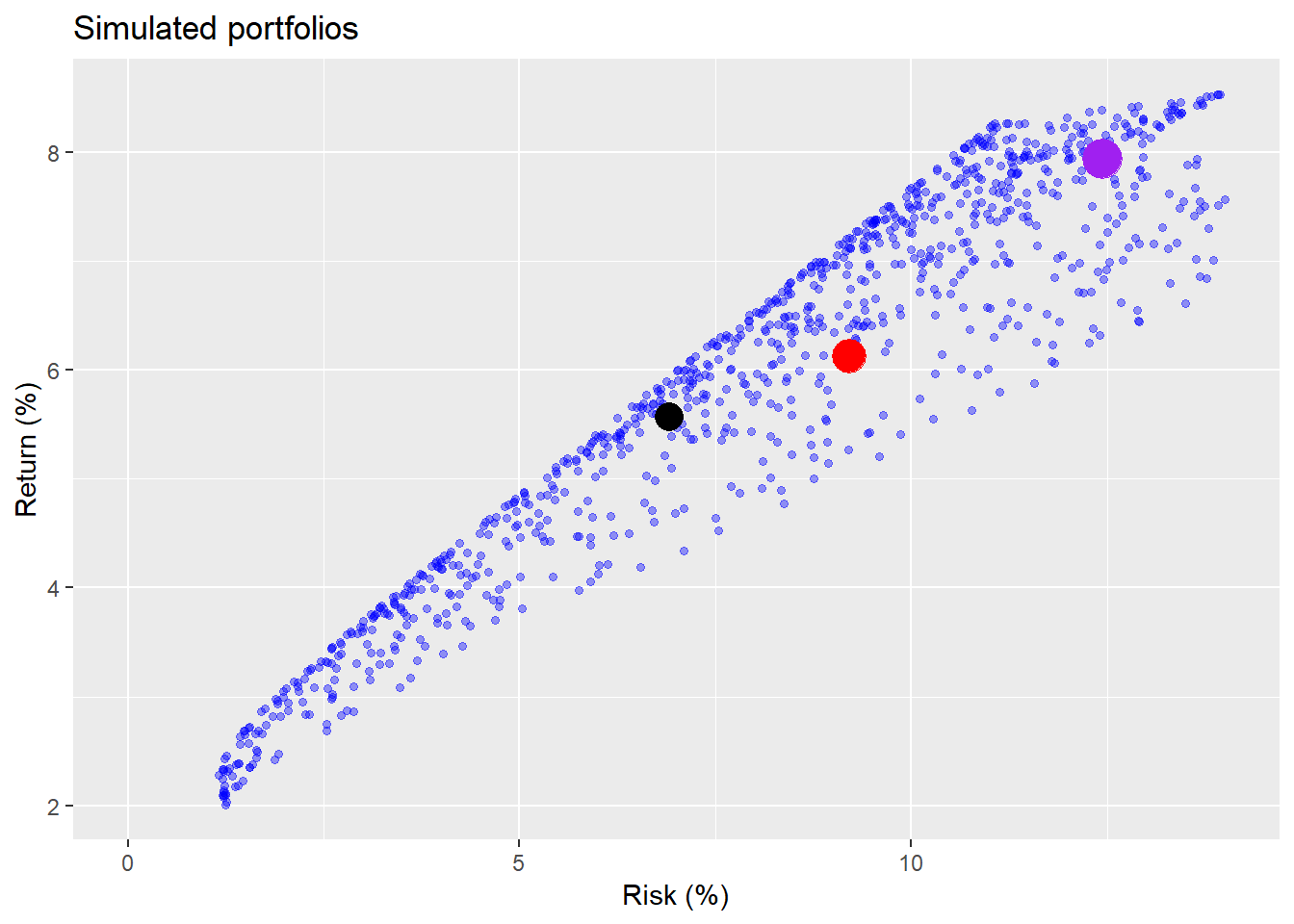However, we showed that some of these dominant portfolios might not be intuitively acceptable even if mathematically optimal. For example, when our hero thought that he was fine with the current riskiness of his portfolio, but wanted to eke out a bit more return, the solution was to increase his exposure to stocks by over 20 points and increase his exposure to gold by four points, all at the expense of bonds. But this only resulted in a one-to-two point improvement in returns. If he wanted to improve returns more than that, he would have to alter how much risk he would be willing to accept.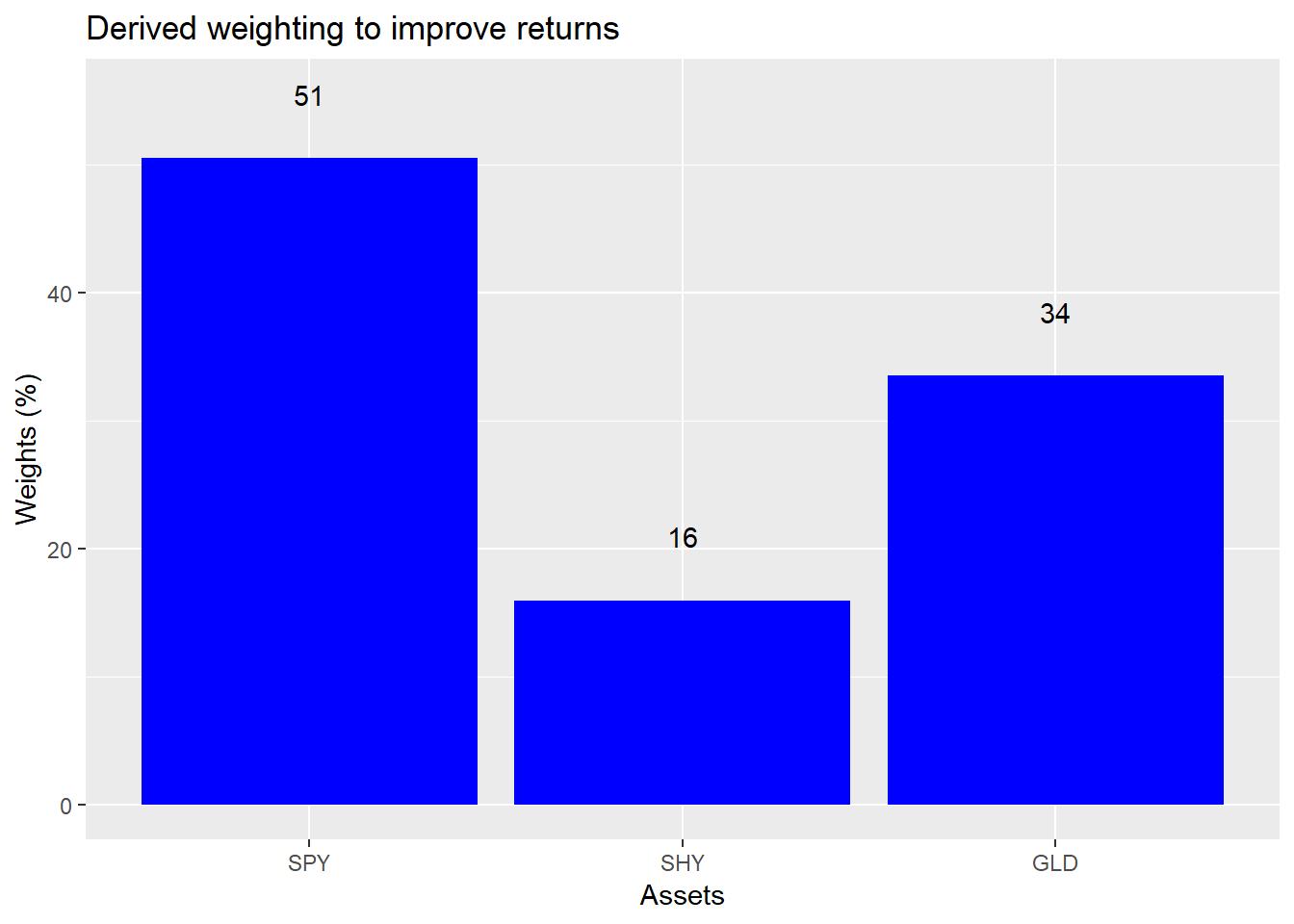That begged the question of whether there was an alternative solution. Let’s resume where we left off…

Now that we’ve seen that a major change in the portfolio weights doesn’t yield that much improvement in returns, should we find a different metric? Maybe we should be looking for the best risk-adjusted return. Let’s graph the same random portfolios, but color them according to their risk-adjusted returns—in this case, simply return over risk—and we’ll call this the Sharpe ratio after the Nobel Prize winner William F. Sharpe who developed the concept.1 The higher the Sharpe ratio the greener the point, the lower the redder.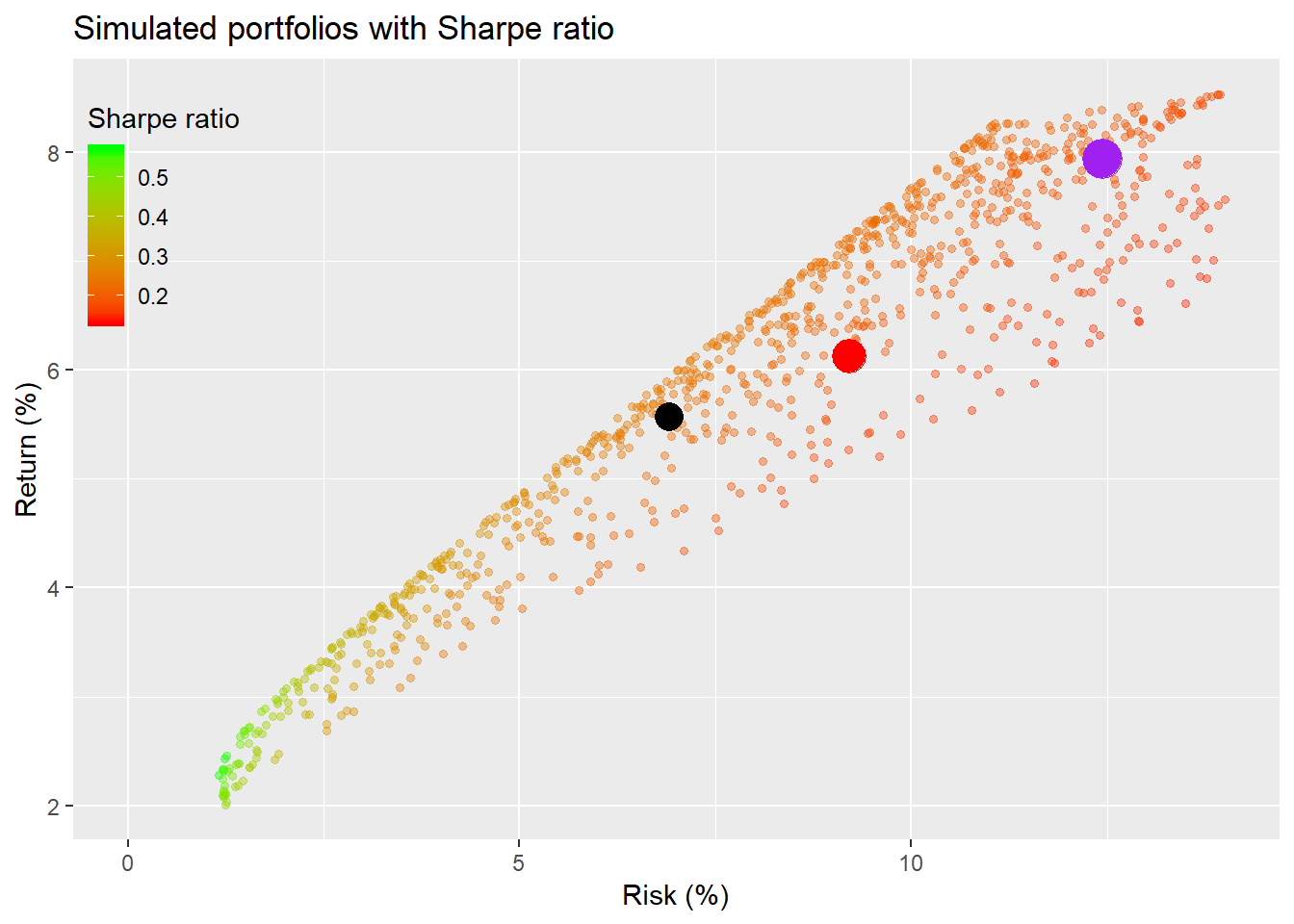Interestingly, the highest risk-adjusted returns appear to be at the low end of the graph. In fact, the highest risk-adjusted return also happens to be the portfolio with the lowest risk. That isn’t exactly counter-intuitive. But it raises the question of how much additional return you’re getting for taking on more risk. To see this we add a line whose slope matches a one-to-one correspondence between change in risk and change in return. This is shown in the graph below.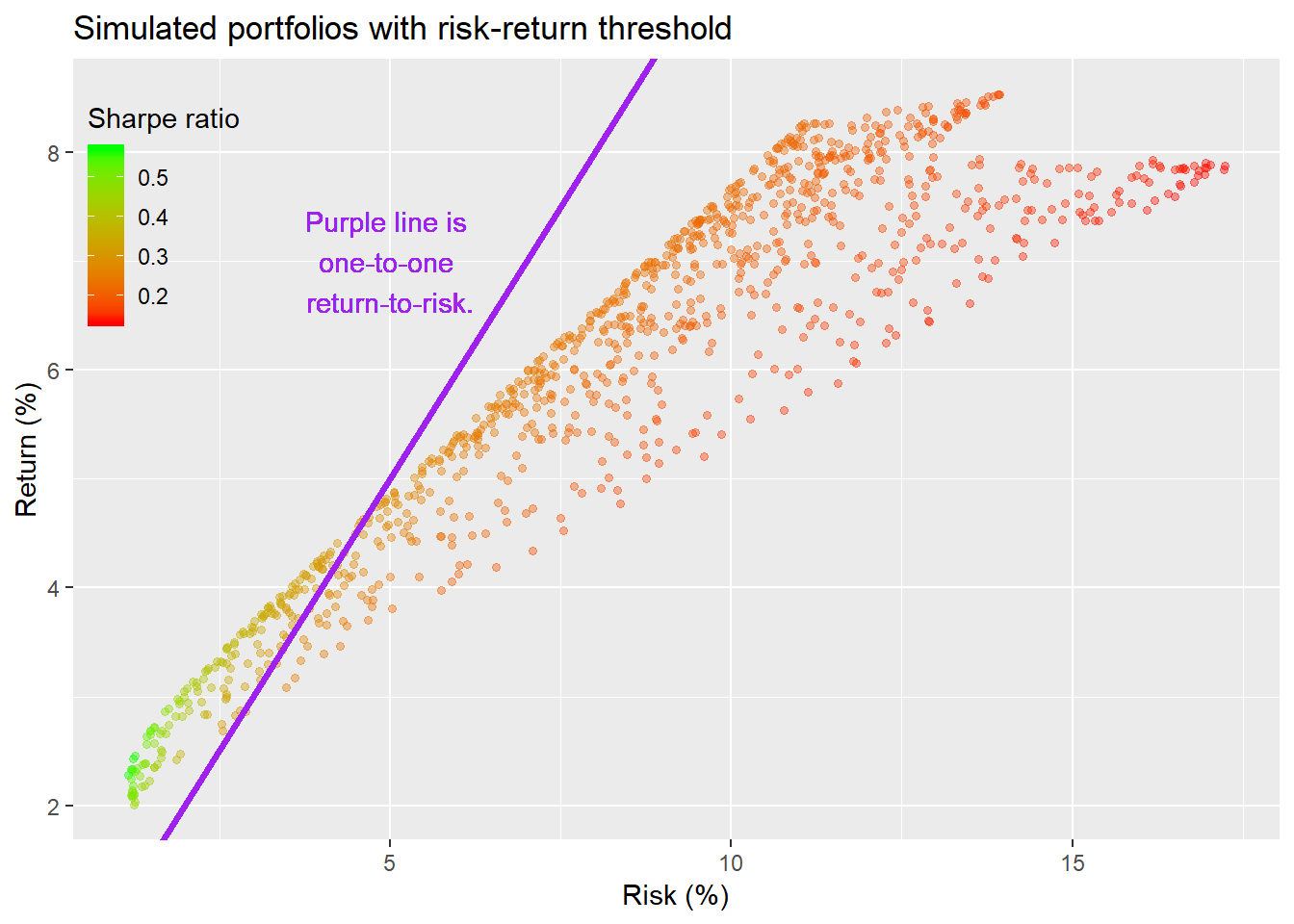What’s interesting about this line is that it tells you which portfolios generate more than one unit of return per unit of risk and which ones generate less. Let’s spend a few moments on the graph.

The points that lie above the purple line represent portfolios where you’re return per unit of risk is greater than one for one. The portfolios below that are, obviously, the opposite. It’s important to remember that in this case, a unit of risk is not the same as a unit of return. Volatility (or the standard deviation of returns) is used as a proxy for risk. Hence, risk is a standardized range, while return is a point. So it’s not the same as risk a dollar to make a dollar. You’re risking a likely range of dollars to make a dollar. If one of the portfolios has a 5% average return and a 10% risk, that means the returns of the portfolio could be -5% to 15% close to 70% of the time. Hence, when risk increases by one unit, the range of possible outcomes widens by two units. In the previous example, the range of values (-5% to 15%) based on risk was about 20 percentage points. If that risk increased by one unit to 11%, then the range would be 22 percentage points (-6% to 16%).

For those not indoctrinated by portfolio theory this isn’t the most intuitive concept on first blush. But think about it this way: embedded in that range of potential values is a risk of loss. By bearing that potential loss, you’re expecting a potential gain. So the purple line cuts the portfolios between those for which the expected upside potential is greater than overall potential. In other words, the upside is greater than a reasonable expectation of the downside and vice versa. Most folks prefer the upside to be greater than the down. In future posts, we’ll re-arrange this to look at risk only as expected loss. But we need to walk before we can run.

Let’s go back and see where the three portfolios are in relation to the purple line.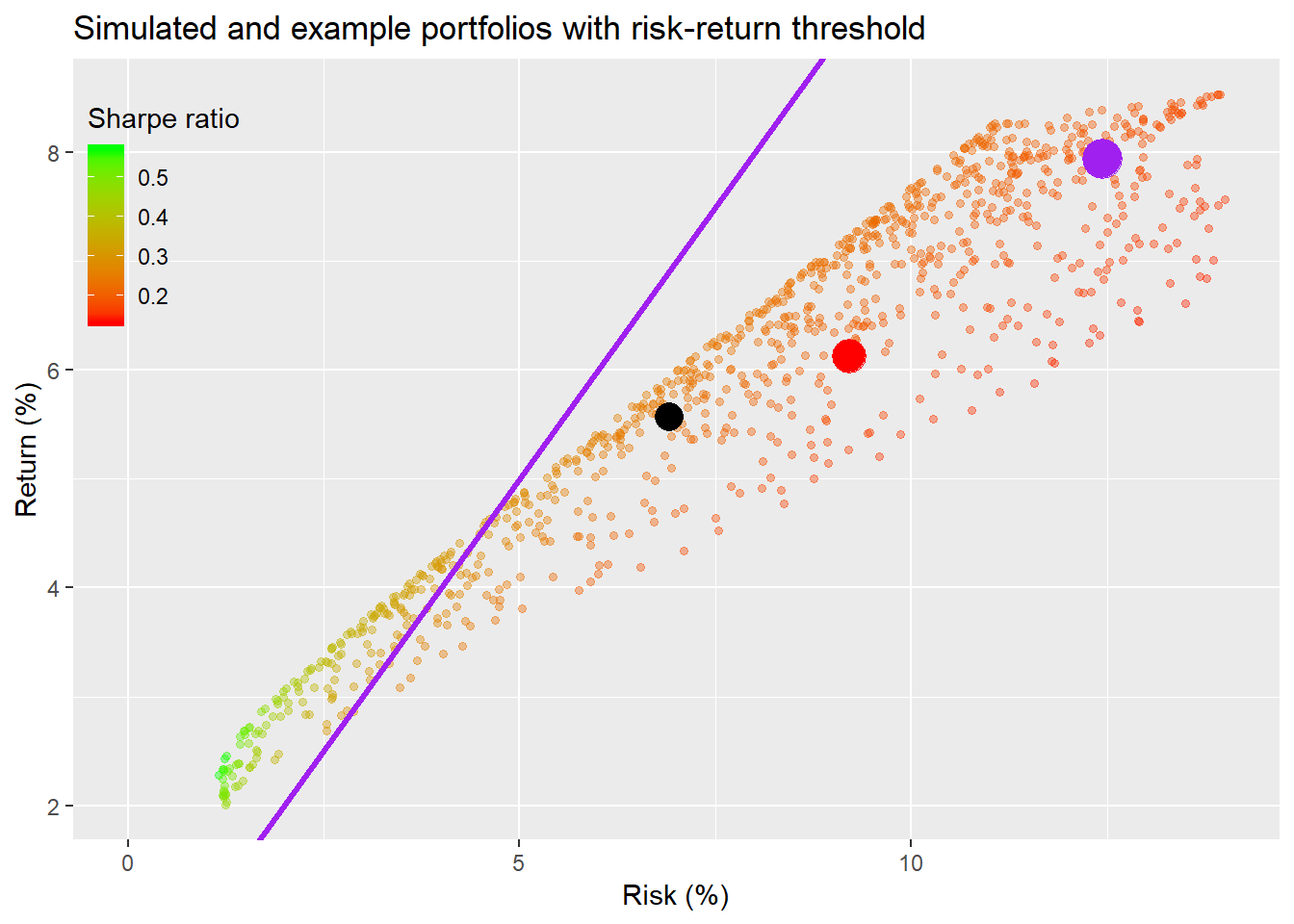None of the portfolios enjoy a one-to-one relationship between return and risk. That doesn’t mean they’re “bad” portfolios. If you’re required return is greater than 5%—roughly the point above which return starts to lag risk—then to achieve that you’ll need to accept a poorer risk-adjusted return profile. That begs the question of whether this trade-off of accepting incrementally more downside potential for incrementally less upside potential is worth it.

Answering the “worth it” question takes us out of the realm of numbers and into the realm of preferences, psychology, and behavior. We won’t dwell too long on this because it’s hard to generalize individual preferences. Behavioral finance attempts to identify and explain the motivation and effect of such preferences. But that is way beyond the scope of this post.

Let’s move on to look at what the average weights for those greater than one-for-one return-to-risk portfolios actually look like.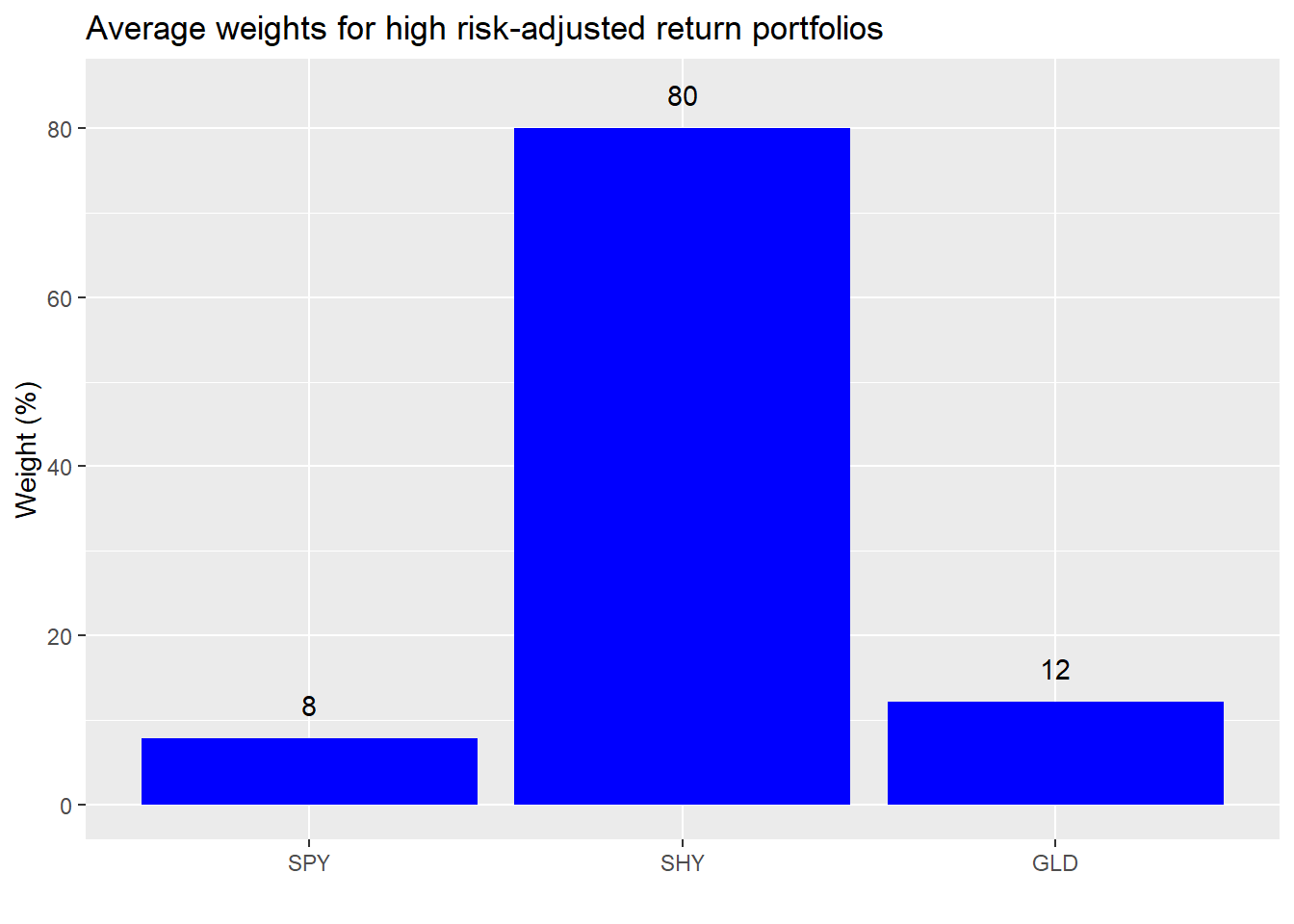On average we see a very high allocation to bonds and not much to stocks or gold. For people that don’t require a high return, this would probably be a good portfolio mix. But let’s assume our protagonist needs more than that, yet he doesn’t want to stray too far from a relatively evenly balanced weighting. So we’ll keep close to the same volatility and see what types of returns we can generate along with the implied portfolio weights. Here’s the original table of returns and risk.

Table 1: Annualized performance metrics
Asset Return (%) Risk (%) Sharpe ratio
Equal 6.0 9.2 0.66
Naive 6.0 6.9 0.81
Risky 8.4 12.4 0.64

Let’s look at the portfolios between the two bands that represent one percentage point more or less risk than the equal-weighted portfolio in the graph below.Now we’ll see what the average returns and risk are for those risk bands. One thing should stand out: while both average returns and risk are higher, so is the Sharpe ratio. In general, then, our hero can achieve better returns and risk-adjusted returns by widening his risk parameters.

Table 2: Average returns and risk for risk bands (%)
Returns Risk Sharpe
7.4 9.7 0.76

That doesn’t seem too bad. Let’s graph the average weights.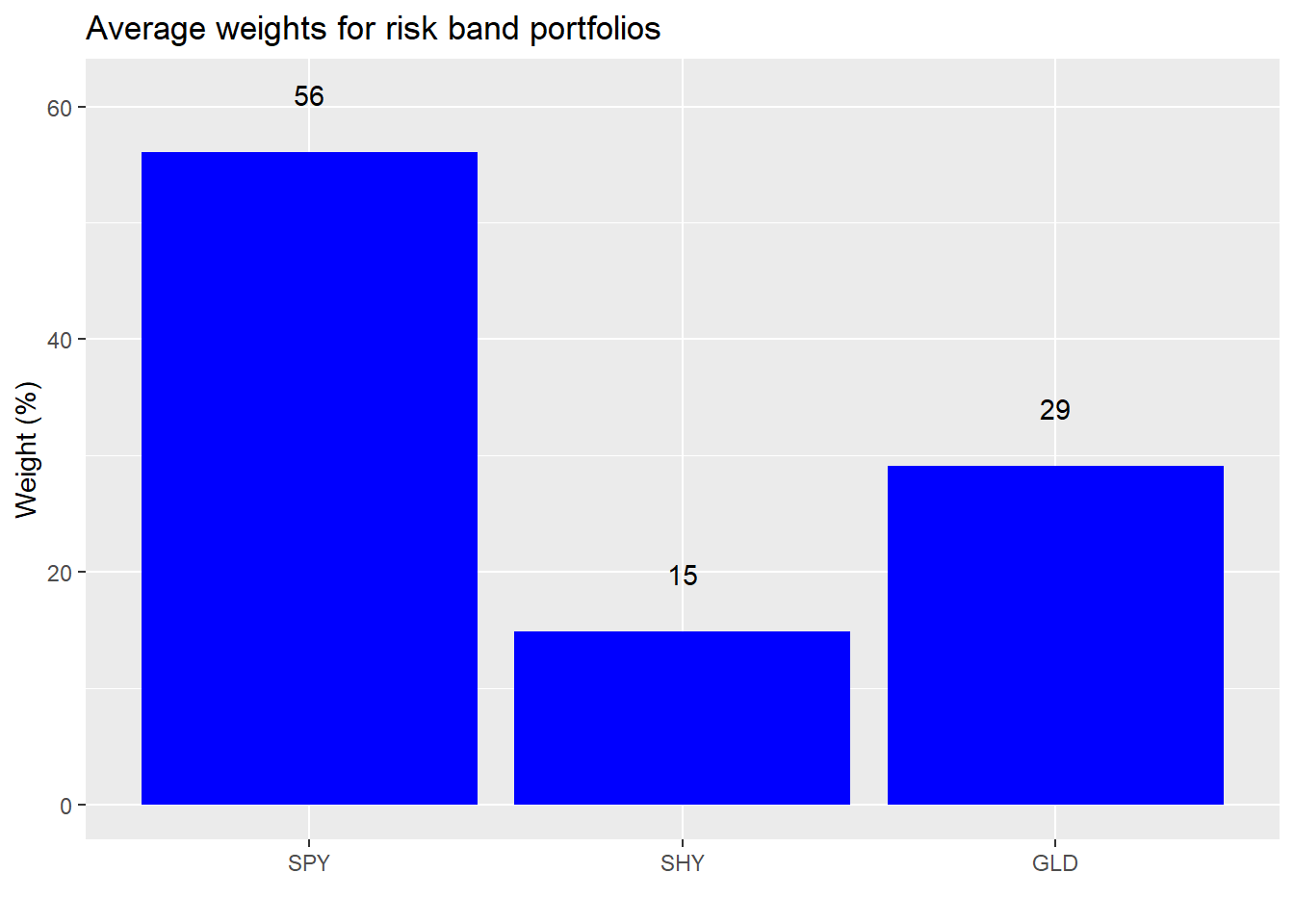Seems reasonable. But you’ll note that this change in allocation isn’t too different from switching to the dominant portfolio we calculated earlier. So our hero thinks that maybe the gold allocation is too high. He wants to see if there are any portfolios that would afford him a similar risk and return, but allocate no more than 20% to gold. Indeed, there is as shown below.

Table 3: Average returns and risk for risk bands with gold constraint (%)
Returns Risk Sharpe
7.3 9.7 0.75

However, to get gold below 20%, we need to raise the allocation to stocks to over 60%. Our hero’s not sure if this is the type of allocation he wants, so he asks if it’s possible to lower the exposure to stocks a little. Unfortunately, no luck there. So what does the average weighting look like?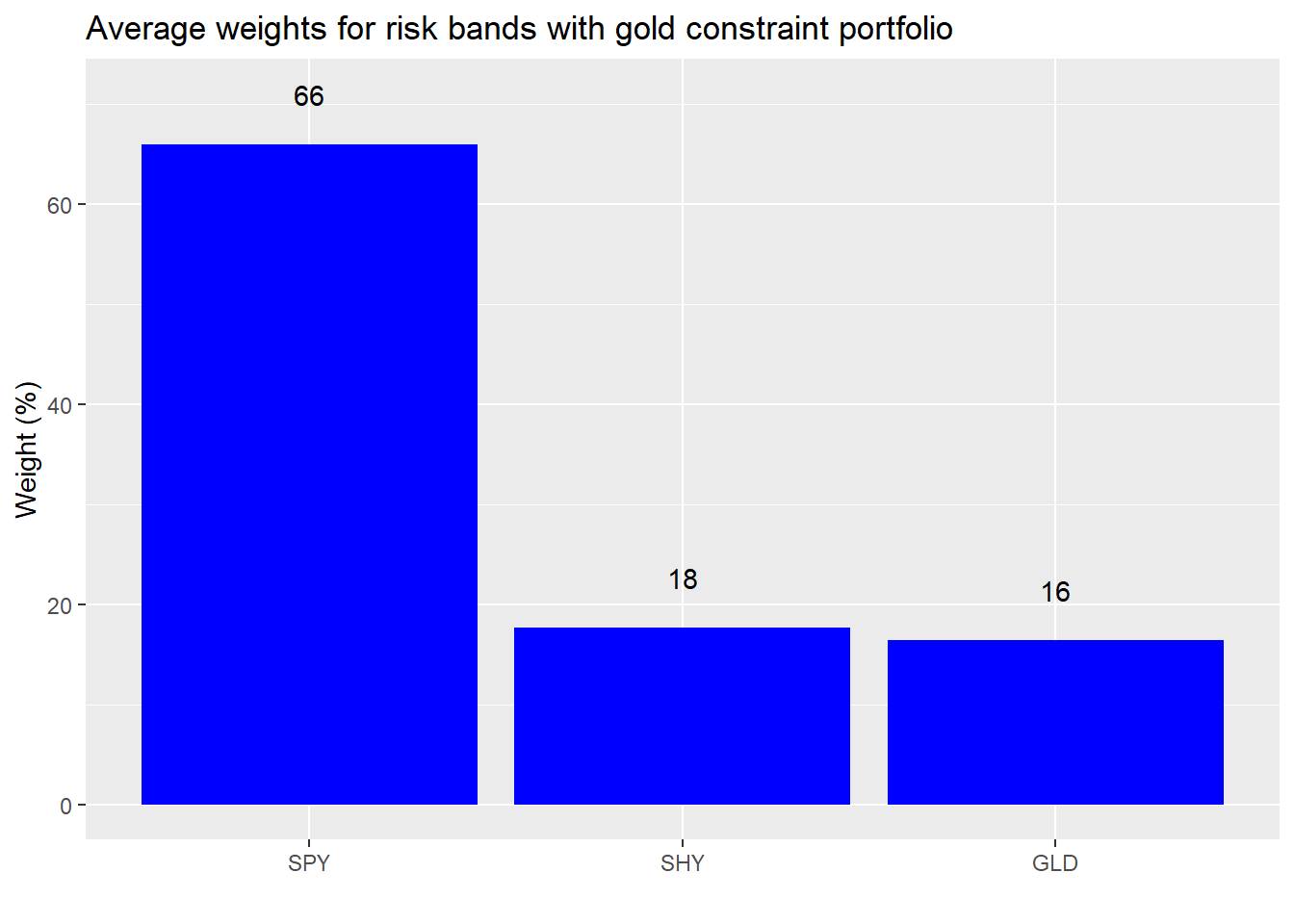Almost two-thirds of the portfolio is allocated to stocks and the remainder is relatively evenly divided among bonds and commodities. Is this acceptable? The weighting to stocks more than doubles, the weighting to gold is almost chopped in half, and the Sharpe ratio improves by over 10%. Our hero may not like the higher weighting to stocks, but at least his risk-adjusted return is much better. Only our hero can tell if he’s comfortable with the new portfolio. Whatever the case, we’re far off from an “optimal” portfolio. Where does the “average” portfolio lie on the scatter plot? The yellow dot is that portfolio, we’ll call it the “sufficient portfolio.”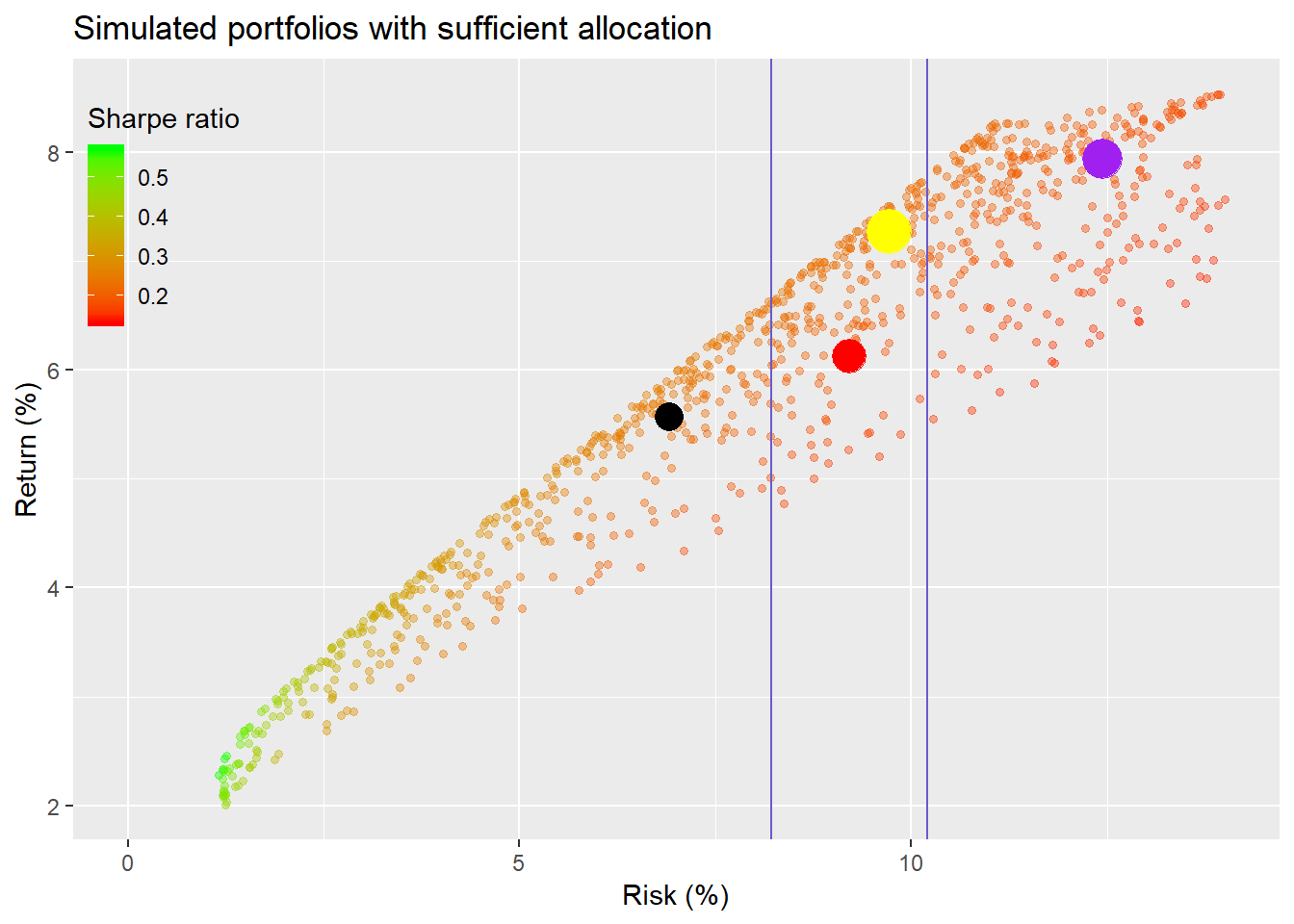What does this tell us? While the sufficient portfolio doesn’t offer the highest return for the given level of risk, it does offer a higher return for only a moderate increase of risk and with an allocation our hero may prefer relative to his cousin’s, co-worker’s, or the remainder of options. But then again it might not, in which case, we’d have to re-run the calculations with different weight constraints. Let’s at least look at how the sufficient would have performed historically, as shown in the graph below with the wider line in purple, before we summarize.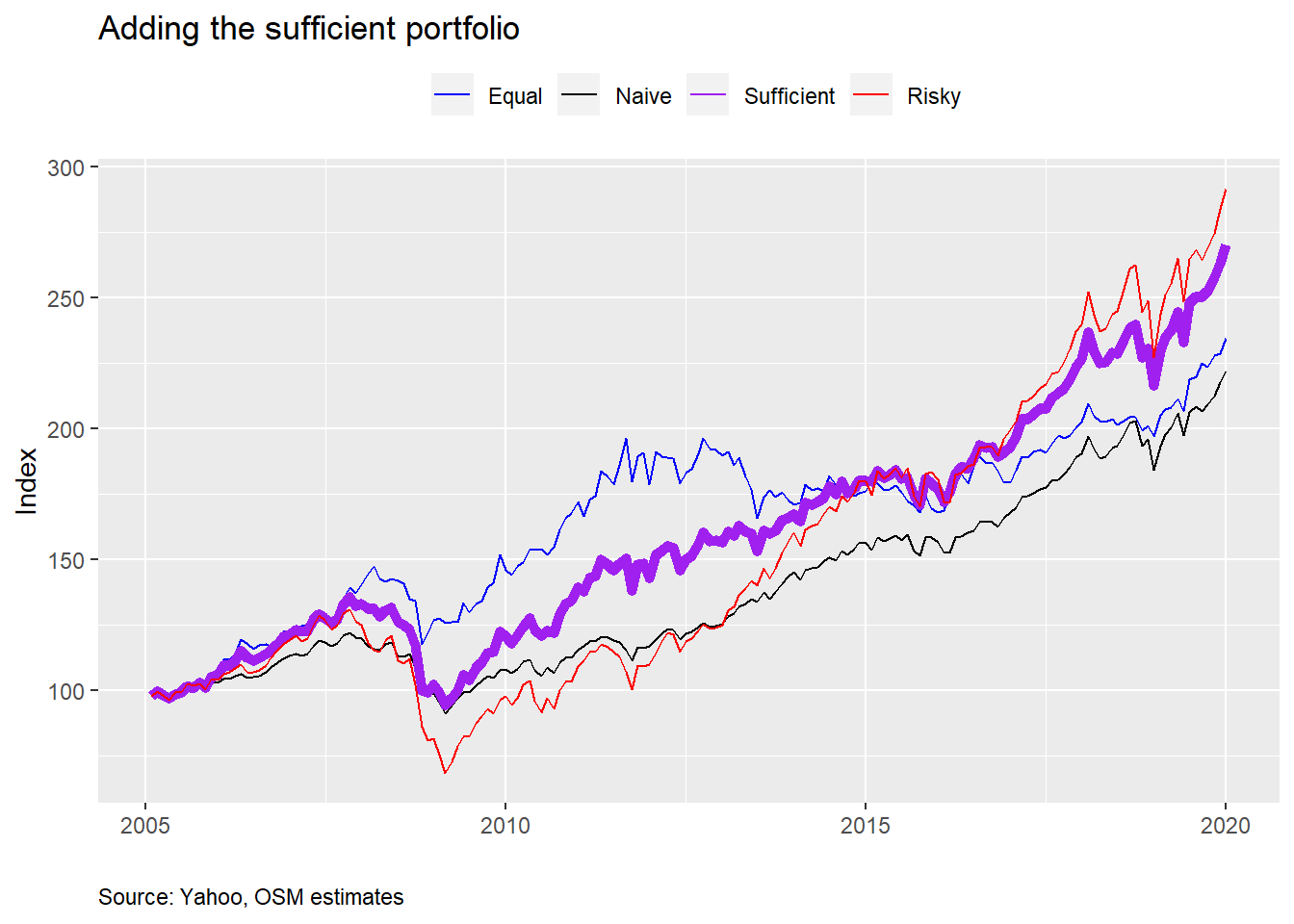What’s the key takeaway? Portfolios that offer the highest return for a given level of risk may not be an allocation that many investors would be comfortable with. And the highest risk-adjusted return portfolio may not offer the required return. But it is possible to find a portfolio that offers most of the necessary requirements and improves risk-adjusted returns if the range of acceptable outcomes is broadened and the constraints aren’t overly stringent. Finding these portfolios becomes more of an iterative process than a closed form solution. Would the new portfolio be satisfactory? At the very least, that depends on the cost of adherence. If the psychological cost to maintain the portfolio is low—that is, it doesn’t keep you up at night—then, provided the portfolio satisfies the other requirements, it is a more “satisfactory” portfolio. This obviously touches on behavioral elements that would require separate posts, but the goal is to view most of these concepts through the lens of what has an intuitive appeal. Eventually, we might find a portfolio that satisfices our hero’s risk and return requirements. That is, it satisfies his needs based on sufficient thresholds. We won’t delve into that concept more in this post, but it will underlie the rest of this series on portfolio construction.

And speaking of this series, there’s still more ground to cover. Over the next few posts we’ll examine benchmarking, rebalancing, semi-deviation, capital market expectations, and time dependence. Stick with us and let us know if there’s something you’d like to see. Until then, here’s the code:

# Load package
library(tidyquant)

# Get data
symbols <- c("SPY", "EEM", "SHY", "IYR", "GLD")
symbols_low <- tolower(symbols)

prices <- getSymbols(symbols, src = "yahoo",
from = "1990-01-01",
auto.assign = TRUE) %>%
reduce(merge) %>%
colnames<-(symbols_low)

prices_monthly <- to.monthly(prices, indexAt = "last", OHLC = FALSE)
ret <- ROC(prices_monthly)["2005/2019"]
naive <- ret[,c("spy", "shy")]
basic <- ret[,c("spy", "shy", "gld")]

# Create different weights and portflios
wt1 <- rep(1/(ncol(basic)), ncol(basic))
port1 <- Return.portfolio(basic, wt1) %>%
colnames<-("ret")

wt2 <- c(0.9, 0.10, 0)
port2 <- Return.portfolio(basic, weights = wt2) %>%
colnames<-("ret")

wtn <- c(0.5, 0.5)
portn <- Return.portfolio(naive, wtn)

port_comp <- data.frame(date = index(port1), equal = as.numeric(port1),
wtd = as.numeric(port2),
naive = as.numeric(portn))

port_comp %>%
gather(key,value, -date) %>%
group_by(key) %>%
mutate(value = cumprod(value+1)) %>%
ggplot(aes(date, value*100, color = key)) +
geom_line() +
scale_color_manual("", labels = c("Equal", "Naive", "Risky"),
values = c("blue", "black", "red")) +
labs(x = "",
y = "Index",
title = "Three portfolios, which is best?",
caption = "Source: Yahoo, OSM estimates") +
theme(legend.position = "top",
plot.caption = element_text(hjust = 0))

# Portfolio
mean_ret <- apply(ret[,c("spy", "shy", "gld")],2,mean)
cov_port <- cov(ret[,c("spy", "shy", "gld")])
port_exam <- data.frame(ports = colnames(port_comp)[-1],
ret = as.numeric(apply(port_comp[,-1],2, mean)),
vol = as.numeric(apply(port_comp[,-1], 2, sd)))

# Weighting that ensures more variation and random weighthing to stocks
set.seed(123)
wts <- matrix(nrow = 1000, ncol = 3)

for(i in 1:1000){
a <- runif(1,0,1)
b <- c()
for(j in 1:2){
b[j] <- runif(1,0,1-sum(a,b))
}
if(sum(a,b) < 1){
inc <- (1-sum(a,b))/3
vec <- c(a+inc, b+inc)
}else{
vec <- c(a,b)
}
wts[i,] <- sample(vec,replace = FALSE)
}

# Calculate random portfolios
port <- matrix(nrow = 1000, ncol = 2)
for(i in 1:1000){
port[i,1] <- as.numeric(sum(wts[i,] * mean_ret))
port[i,2] <- as.numeric(sqrt(t(wts[i,] %*% cov_port %*% wts[i,])))
}

colnames(port) <- c("returns", "risk")
port <- as.data.frame(port)

# Graph with points
port %>%
ggplot(aes(risk*sqrt(12)*100, returns*1200)) +
geom_point(color = "blue", size = 1.2, alpha = 0.4) +
geom_smooth(method = "loess", formula = y ~ log(x), se = FALSE, color = "slategrey") +
geom_point(data = port_exam, aes(port_exam[1,3]*sqrt(12)*100,
port_exam[1,2]*1200),
color = "red", size = 6) +
geom_point(data = port_exam, aes(port_exam[2,3]*sqrt(12)*100,
port_exam[2,2]*1200),
color = "purple", size = 7) +
geom_point(data = port_exam, aes(port_exam[3,3]*sqrt(12)*100,
port_exam[3,2]*1200),
color = "black", size = 5) +
scale_x_continuous(limits = c(0,14)) +
labs(x = "Risk (%)",
y = "Return (%)",
title = "Simulated portfolios")

# Finad max and equivalent risk for Equal risk slice
equal_max <- port %>%
filter(risk < port_exam[1,3]+0.0005,
risk > port_exam[1,3]-0.0005) %>%
mutate(returns = returns*1200,
risk = risk * sqrt(12)*100) %>%
arrange(desc(returns)) %>%
slice(1)

# Find wieghts for dominant portfolio
eq_wt <- port %>%
mutate(spy_wt = wts[,1],
shy_wt = wts[,2],
gld_wt = wts[,3],
returns = returns * 1200,
risk = risk * sqrt(12) *100) %>%
filter(returns == equal_max$returns, risk == equal_max$risk) %>%
select(spy_wt, shy_wt, gld_wt)

# Graph weights
eq_wt %>%
rename("SPY" = spy_wt,
"SHY" = shy_wt,
"GLD" = gld_wt) %>%
gather(key,value) %>%
ggplot(aes(factor(key, level = c("SPY", "SHY", "GLD")), value*100)) +
geom_bar(stat = 'identity', fill = "blue") +
geom_text(aes(label = round(value,2)*100), nudge_y = 5) +
labs(x = "Assets",
y = "Weights (%)",
title = "Derived weighting to improve returns")

# Portfolio with Sharpe ratio
port %>%
ggplot(aes(risk*sqrt(12)*100, returns*1200, color = sharpe)) +
geom_point(size = 1.2, alpha = 0.4) +
geom_point(data = port_exam, aes(port_exam[1,3]*sqrt(12)*100,
port_exam[1,2]*1200),
color = "red", size = 6) +
geom_point(data = port_exam, aes(port_exam[2,3]*sqrt(12)*100,
port_exam[2,2]*1200),
color = "purple", size = 7) +
geom_point(data = port_exam, aes(port_exam[3,3]*sqrt(12)*100,
port_exam[3,2]*1200),
color = "black", size = 5) +
scale_x_continuous(limits = c(0,14)) +
labs(x = "Risk (%)",
y = "Return (%)",
title = "Simulated portfolios",
color = "Sharpe ratio") +
scale_color_gradient(low = "red", high = "green") +
theme(legend.position = "top", legend.key.size = unit(.5, "cm"))

# Portfolio with sharpe line
max_sharpe <- max(port\$sharpe)*sqrt(12)
port %>%
ggplot(aes(risk*sqrt(12)*100, returns*1200, color = sharpe)) +
geom_point(size = 1.2, alpha = 0.4) +
geom_abline(intercept = 0, slope = max_sharpe, color = "blue") +
labs(x = "Risk (%)",
y = "Return (%)",
title = "Simulated portfolios",
color = "Sharpe ratio") +
scale_color_gradient(low = "red", high = "green") +
theme(legend.position = "top", legend.key.size = unit(.5, "cm"))

# Graph with one-to-one
port %>%
ggplot(aes(risk*sqrt(12)*100, returns*1200, color = sharpe)) +
geom_point(size = 1.2, alpha = 0.4) +
geom_abline(intercept = 0, slope = max_sharpe, color = "blue") +
geom_abline(color = "purple", lwd = 1.25)+
labs(x = "Risk (%)",
y = "Return (%)",
title = "Simulated portfolios",
color = "Sharpe ratio") +
scale_color_gradient(low = "red", high = "green") +
geom_text(aes(x = 5, y = 7),
label = "Purple line is \none-to-one \nreturn-to-risk.",
color = "purple")

# Three portfolios with purple line
port %>%
ggplot(aes(risk*sqrt(12)*100, returns*1200, color = sharpe)) +
geom_point(size = 1.2, alpha = 0.4) +
geom_point(data = port_exam, aes(port_exam[1,3]*sqrt(12)*100,
port_exam[1,2]*1200),
color = "red", size = 6) +
geom_point(data = port_exam, aes(port_exam[2,3]*sqrt(12)*100,
port_exam[2,2]*1200),
color = "purple", size = 7) +
geom_point(data = port_exam, aes(port_exam[3,3]*sqrt(12)*100,
port_exam[3,2]*1200),
color = "black", size = 5) +
geom_abline(color = "purple", size = 1.1) +
scale_x_continuous(limits = c(0,14)) +
labs(x = "Risk (%)",
y = "Return (%)",
title = "Simulated portfolios",
color = "Sharpe ratio") +
scale_color_gradient(low = "red", high = "green")

port %>%
mutate(SPY = wts[,1],
SHY = wts[,2],
GLD = wts[,3],
returns = returns * 1200,
risk = risk * sqrt(12) *100,
sharpe = sharpe*sqrt(12)) %>%
filter(sharpe >= 1) %>%
summarise_all(mean) %>%
gather(key, value) %>%
filter(!key %in% c("returns", "risk", "sharpe")) %>%
ggplot(aes(factor(key, labels = c("SPY", "SHY", "GLD")), value *100)) +
geom_bar(stat = "identity", fill = "blue") +
labs(x = "",
y = "Weight (%)",
title = "Average weights for high risk-adjusted return portfolios") +
geom_text(aes(label = round(value,2)*100), nudge_y = 4)

# Table
port_comp %>%
rename("Equal" = equal,
"Naive" = naive,
"Risky" = wtd) %>%
gather(Asset, value, -date) %>%
group_by(Asset) %>%
summarise(Mean (%) = round(mean(value, na.rm = TRUE),3)*1200,
Volatility (%) = round(sd(value, na.rm = TRUE)*sqrt(12),3)*100,
Risk-adjusted (%) = round(mean(value, na.rm = TRUE)/sd(value, na.rm=TRUE)*sqrt(12),3)*100,
Cumulative (%) = round(prod(1+value, na.rm = TRUE),3)*100) %>%
knitr::kable(caption = "Annualized performance metrics")

# Graph with risk bands
port %>%
ggplot(aes(risk*sqrt(12)*100, returns*1200)) +
geom_point(color = "blue", size = 1.2, alpha = 0.4) +
geom_point(data = port_exam, aes(port_exam[1,3]*sqrt(12)*100,
port_exam[1,2]*1200),
color = "red", size = 6) +
geom_vline(xintercept = up_band, color = "slateblue") +
geom_vline(xintercept = down_band, color = "slateblue") +
labs(x = "Risk (%)",
y = "Return (%)",
title = "Simulated portfolios")

# Portfoilio band output for blog
port %>%
mutate(spy_wt = wts[,1],
shy_wt = wts[,2],
gld_wt = wts[,3],
returns = returns * 1200,
risk = risk * sqrt(12) *100,
sharpe = returns/risk) %>%
filter(returns > port_exam[1,2]*1200 +1,
risk >= down_band,
risk < up_band) %>%
summarise_all(function(x) round(mean(x),1)) %>%
select(returns, risk, sharpe) %>%
rename("Returns" = returns,
"Risk" = risk,
"Sharpe" = sharpe) %>%
knitr::kable(caption = "Average returns and risk for risk bands (%)")

port %>%
mutate(SPY = wts[,1],
SHY = wts[,2],
GLD = wts[,3],
returns = returns * 1200,
risk = risk * sqrt(12) *100,
sharpe = returns/risk) %>%
filter(returns > port_exam[1,2]*1200 +1,
risk >= down_band,
risk < up_band) %>%
summarise_all(mean) %>%
gather(key, value) %>%
filter(key %in% c("SPY", "SHY", "GLD")) %>%
ggplot(aes(factor(key, levels = c("SPY", "SHY", "GLD")) ,value*100)) +
geom_bar(stat = "identity", fill = "blue") +
labs(x = "",
y = "Weight (%)",
title = "Average weights for high risk-adjusted return portfolios") +
geom_text(aes(label = round(value,2)*100), nudge_y = 5)

# Portfoilio band output for blog
port %>%
mutate(spy_wt = wts[,1],
shy_wt = wts[,2],
gld_wt = wts[,3],
returns = returns * 1200,
risk = risk * sqrt(12) *100,
sharpe = returns/risk) %>%
filter(returns > port_exam[1,2]*1200 +1,
risk >= down_band,
risk < up_band,
gld_wt <= 0.2) %>%
summarise_all(function(x) round(mean(x),2)) %>%
select(returns, risk, sharpe) %>%
rename("Returns" = returns,
"Risk" = risk,
"Sharpe" = sharpe) %>%
knitr::kable(caption = "Average returns and risk for risk bands (%)")

# Bar chart of weights
port %>%
mutate(SPY = wts[,1],
SHY = wts[,2],
GLD = wts[,3],
returns = returns * 1200,
risk = risk * sqrt(12) *100,
sharpe = returns/risk) %>%
filter(returns > port_exam[1,2]*1200 +1,
risk >= down_band,
risk < up_band,
GLD <= 0.2) %>%
summarise_all(mean) %>%
gather(key, value) %>%
filter(key %in% c("SPY", "SHY", "GLD")) %>%
ggplot(aes(factor(key, levels = c("SPY", "SHY", "GLD")) ,value*100)) +
geom_bar(stat = "identity", fill = "blue") +
labs(x = "",
y = "Weight (%)",
title = "Average weights for high risk-adjusted return portfolios") +
geom_text(aes(label = round(value,2)*100), nudge_y = 5)

port %>%
ggplot(aes(risk*sqrt(12)*100, returns*1200)) +
geom_point(color = "blue", size = 1.2, alpha = 0.4) +
geom_point(data = port_exam, aes(port_exam[1,3]*sqrt(12)*100,
port_exam[1,2]*1200),
color = "red", size = 6) +
geom_point(data = port_exam, aes(port_exam[2,3]*sqrt(12)*100,
port_exam[2,2]*1200),
color = "purple", size = 7) +
geom_point(data = port_exam, aes(port_exam[3,3]*sqrt(12)*100,
port_exam[3,2]*1200),
color = "black", size = 5) +
geom_point(data = suff_port, aes(risk,returns),
color = "yellow", size = 8) +
geom_vline(xintercept = up_band, color = "slateblue") +
geom_vline(xintercept = down_band, color = "slateblue") +
scale_x_continuous(limits = c(0,14)) +
labs(x = "Risk (%)",
y = "Return (%)",
title = "Simulated portfolios with sufficient allocation")

port_suff <- Return.portfolio(basic,suff_port_wts) %>%
colnames<-("suff")

# Graph
port_comp %>%
mutate(suff = as.numeric(port_suff)) %>%
gather(key,value, -date) %>%
group_by(key) %>%
mutate(value = cumprod(value+1)) %>%
ggplot(aes(date, value*100, color = key)) +
geom_line(aes(size = key)) +
scale_color_manual("", labels = c("Equal", "Naive", "Sufficient", "Risky"),
values = c("blue", "black", "purple","red")) +
scale_size_manual(values = c(1,1,2,1), guide = 'none') +
labs(x = "",
y = "Index",
title = "Adding the sufficient portfolio",
caption = "Source: Yahoo, OSM estimates") +
theme(legend.position = "top",
plot.caption = element_text(hjust = 0))

1. The Sharpe ratio was developed by William Sharpe to measure the excess return of an asset over risk-free rates adjusted for volatility.